# DAV Class 5 Maths Chapter 3 Brain Teasers Solutions

The DAV Class 5 Maths Book Solutions Pdf and DAV Class 5 Maths Chapter 3 Brain Teasers Solutions of Multiples and Factors offer comprehensive answers to textbook questions.

## DAV Class 5 Maths Ch 3 Brain Teasers Solutions

Question 1.
(a) Which of the following is not a factor of 48?
(i) 6
(ii) 7
(iii) 12
(iv) 48
Solution:
Factor of 48
6 × 8 = 48
12 × 4 = 48
48 × 1 = 48
7 × ? = ?

(b) Which of the following is a prime number?
(i) 91
(ii) 57
(iii) 97
(iv) 81
Solution:
A prime number is a number that has only two factors: 1 and the number itself.
91 = 1 × 7 × 13 × 91
57 = 1 × 3 × 19 × 57
97 = 1 × 97
81 = 1 × 3 × 9 × 27 × 81
So 97 has only two factors.(c) The LCM of 10, 20, 25 is
(i) 20
(ii) 50
(iii) 100
(iv) 75
Solution: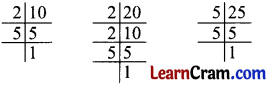Prime factorisation of 10 = 2 × 5
Prime factorisation of 20 = 2 × 2 × 5
Prime factorisation of 25 = 5 × 5
LCM of 10, 20, 25 = 2 × 2 × 5 × 5 = 100

(d) The prime factorisation of 36 is
(i) 4 × 3 × 3
(ii) 2 × 2 × 9
(iii) 12 × 3
(iv) 2 × 2 × 3 × 3
Solution: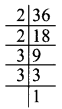Prime factorisation of 36 = 2 × 2 × 3 × 3
So option (iv) is the answer.

(e) 48 has ____ factors.
(i) 10
(ii) 8
(iii) 1
(iv) 6
Solution:
Factors of 48 = 1, 2, 3, 4, 6, 8, 12, 16, 14, 18
Hence, there are 10 factors of 48.
So option (i) is the answer.Question 2.
Write the first four multiples of:
(a) 1
Solution:
7 = 7, 14, 21, 28

(b) 9
Solution:
9 = 9, 18, 27, 36

(c) 12
Solution:
12 = 12, 24, 36, 48

(d) 1
Solution:
1 = 1, 2, 3, 4

(e) 13
Solution:
13 = 13, 26, 39, 52

Question 3.
Fill in the blanks.
(a) 2 × 3 × 7 = 42; 42 is a multiple of ____, _____, and _____.
Solution:
2, 3 and 7

(b) Fifth multiple of 9 : ______
Solution:
45

(c) Is 48 a multiple of 6? _______ (Yes/No)
Solution:
Yes

(d) Least multiple of 65 is ________
Solution:
65

(e) 7 × 3 = 21 : ______ and _____ are factors of _______.
Solution:
7, 3, 21

(f) Is 8 a factor of 70? ______ (Yes/No)
Solution:
No

(g) Is 1 a prime number? ______ (Yes/No)
Solution:
NoQuestion 4.
Find the factors of the following:
(a) 27
Solution:
27 = 1, 3, 9, 27

(b) 90
Solution:
90 = 1, 2, 3, 5, 6, 9, 10, 15, 18, 30, 45, 90

(c) 38
Solution:
38 = 1, 2, 19, 38

(d) 40
Solution:
40 = 1, 2, 4, 5, 8, 10, 20, 40

Question 5.
Is 217 divisible by 27?
Solution:
No, 217 is not divisible by 27.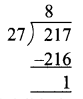Question 6.
Using Factor Tree Method, find the prime factorisation of the following:
(a) 30
Solution: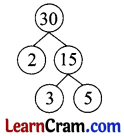Prime factorisation of 30 = 2 × 3 × 5

(b) 84
Solution: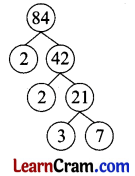Prime factorisation of 84 = 2 × 2 × 3 × 7Question 7.
Using Division Method, find the prime factorisation of the following:
(a) 36
Solution: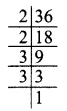Prime factorisation of 36 = 2 × 2 × 3 × 3

(b) 74
Solution:Prime factorisation of 74 = 2 × 37

Question 8.
Find the LCM of:
(a) 28 and 42
Solution: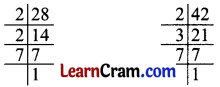Prime factorisation of 28 = 2 × 2 × 7
Prime factorisation of 42 = 2 × 3 × 7
LCM of 28 and 42 = 2 × 2 × 3 × 7 = 84

(b) 10 and 95
Solution:Prime factorisation of 10 = 2 × 5
Prime factorisation fo 95 = 5 × 19
LCM of 10 and 5 = 2 × 5 × 19 = 190Question 9.
How many even numbers are there between 20 and 50?
Solution:
22, 24, 26, 28, 30, 32, 34, 36, 38, 40, 42, 44, 46, 48
even numbers between 20 and 50 = 14 numbers

Question 10.
Write all prime numbers between 50 and 80.
Solution:
Prime number between 50 and 80 = 53, 59, 61, 67, 71, 73, 79.

Question 11.
Write any five odd multiples of 3.
Solution:
5 odd multiples of 3 = 3, 9, 15, 21, 27

Question 1.
Write the next five multiples of the number given below:
(a) 10, 20, 30, ____, ____, ____, ____, ____,
Solution:
40, 50, 60, 70, 80

(b) 12, 24, 36, ____, ____, ____, ____, ____,
Solution:
48, 60, 72, 84, 96

(c) 13, 26, 39, ____, ____, ____, ____, ____,
Solution:
54, 65, 78, 91, 104

(d) 17, 34, 51, ____, ____, ____, ____, ____,
Solution:
68, 85, 102, 119, 136

(e) 19, 38, 57, ____, ____, ____, ____, ____,
Solution:
76, 95, 114, 133, 152Question 2.
Find the LCM in each case.
(a) 14, 35
Solution:
14, 35Prime factorisation of 14 = 2 × 7
Prime factorisation of 35 = 5 × 7
LCM of 14 and 35 = 7

(b) 8, 10, 20
Solution:
8, 10, 20Prime factorisation of 8 = 2 × 2 × 2
Prime factorisation of 10 = 2 × 5
Prime factorisation of 20 = 2 × 2 × 5
LCM of 8, 10 and 20 = 2

(c) 15, 35, 45
Solution:
15, 35, 45Prime factorisation of 15 = 3 × 5
Prime factorisation of 35 = 5 × 7
Prime factorisation of 45 = 3 × 3 × 5
LCM of 15, 35 and 45 = 5

Question 3.
Write all the factors of the following numbers.
(a) 57
Solution:
Factors of 57 = 1 × 3 × 19 × 57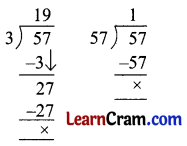(b) 218
Solution:
Factors of 218 = 1 × 2 × 109 × 218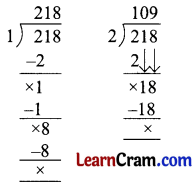(c) 100
Solution:
Factors of 100 = 1 × 2 × 5 × 10 × 25 × 50 × 100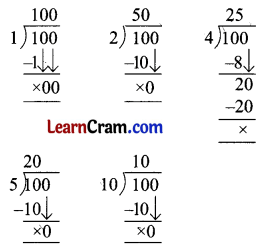(d) 105
Solution:
Factors of 105 = 1 × 3 × 5 × 21 × 35 × 105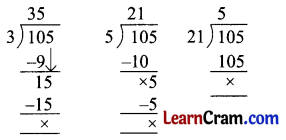Question 4.
Find the HCF of the following:
(a) 15, 36, 50
Solution:
15, 36, 50
Factors of 15 = 1 × 3 × 5 × 15
Factors of 36 = 1 × 2 × 3 × 4 × 9 × 18 × 12 × 36
Factors of 50 = 1 × 2 × 5 × 10 × 25 × 50
Common factors of 15, 36, and 50 = 1
HCF of 15, 36 and 50 = 1

(b) 10, 12, 20
Solution:
10, 12, 20
Factors of 10 = 1 × 2 × 5 × 10
Factors of 12 = 1 × 2 × 3 × 6 × 4 × 12
Factors of 20 = 1 × 2 × 5 × 4 × 10 × 20
Common factors of 10, 12, and 20 = 1, 2
HCF of 10, 12, and 20 = 2

(c) 25, 30, 45
Solution:
25, 30, 45
Factors of 25 = 1 × 5 × 25
Factors of 30 = 1 × 2 × 3 × 5 × 6 × 10 × 15 × 30
Factors of 45 = 1 × 3 × 5 × 9 × 15 × 45
Common factors of 25, 30 and 45 = 1, 5
HCF of 25, 30 and 45 = 5

Question 5.
Circle the composite number.
9, 7, 11, 22, 32, 33, 40, 10, 17, 27, 50, 19, 21, 20, 25, 42, 52, 47
Solution:
9, 22, 32, 33, 40, 10, 27, 50, 21, 20, 25, 42, 52

Question 6.
Using the division method, find the prime factorisation of the following:
(a) 35
Solution:
35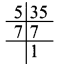Prime factorisation of 35 = 5 × 7

(b) 21
Solution:
21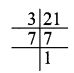Prime factorisation of 21 = 3 × 7

(c) 82
Solution:
82Prime factorisation of 82 = 2 × 41

(d) 95
Solution:
95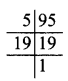Prime factorisation of 95 = 5 × 19

(e) 56
Solution:
56Prime factorisation of 56 = 2 × 2 × 2 × 7Question 7.
Using Factor Tree Method, find the prime factorisation of the following:
(a) 104
Solution:(b) 96
Solution:(c) 112
Solution:(d) 56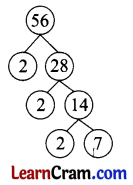Question 8.
Write the prime factorisation of:
(a) 72
Solution:
prime factors of 72 = 2 × 2 × 2 × 3 × 3

(b) 120
Solution:
prime factors of 120 = 2 × 2 × 2 × 3 × 5

(c) 135
Solution:
prime factors of 135 = 3 × 3 × 3 × 5

(d) 145
Solution:
prime factors of 145 = 5 × 29

Question 9.
Find the HCF of the following:
(a) 12, 20, 50
Solution:
2

(b) 24, 72, 90
Solution:
6

(c) 15, 25, 75
Solution:
5

(d) 27, 35, 45
Solution:
1Question 10.
Circle the prime number in the following group:
10, 15, 19, 7, 21, 11, 13, 5, 3, 79, 19, 37, 51, 29, 17, 37, 41, 53, 57
Solution:
19, 7, 11, 13, 3, 79, 19, 37, 29, 17, 37, 41, 53

Question 11.
Using the Prime Factorisation Method, find the LCM.
(a) 20, 40
Solution:
2 × 2 × 5 × 2 = 40

(b) 9, 16, 48
Solution:
2 × 2 × 3 × 2 × 3 × 2 = 144

(c) 15, 75, 60
Solution:
3 × 5 × 5 × 4 = 300

Question 12.
How many even numbers are there between 30 and 70?
Solution:
18

Question 13.
Fill in the blanks: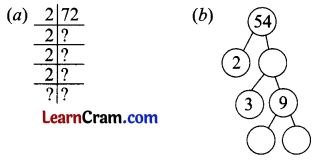Solution: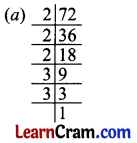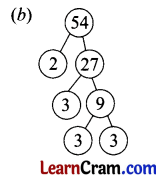Question 14.
Using the Division Method, find the prime factorisation of the following:
(a) 105
Solution:
prime factors of 105 = 3 × 5 × 7

(b) 76
Solution:
prime factors of 76 = 2 × 2 × 19

(c) 95
Solution:
prime factors of 95 = 5 × 19

(d) 102
Solution:
prime factors of 102 = 2 × 3 × 17